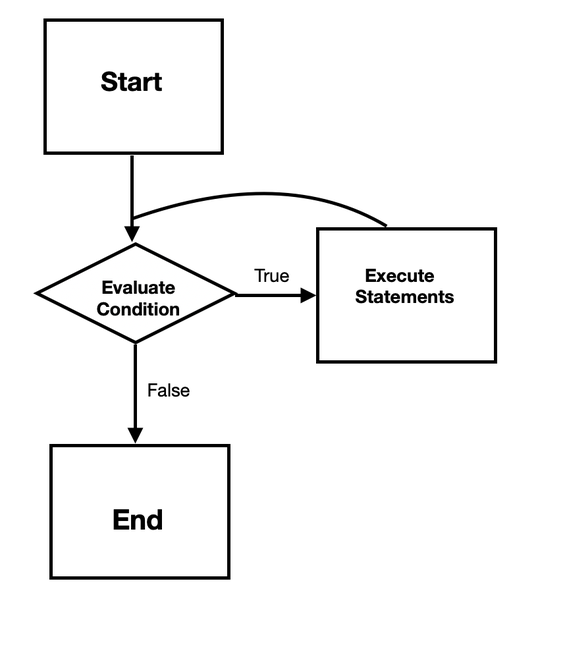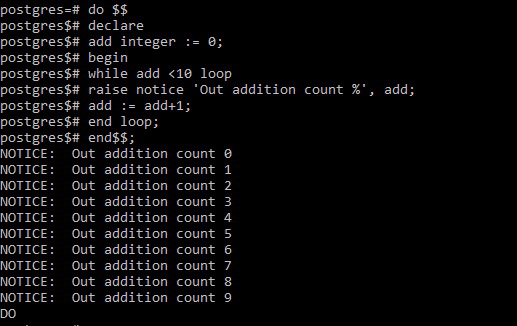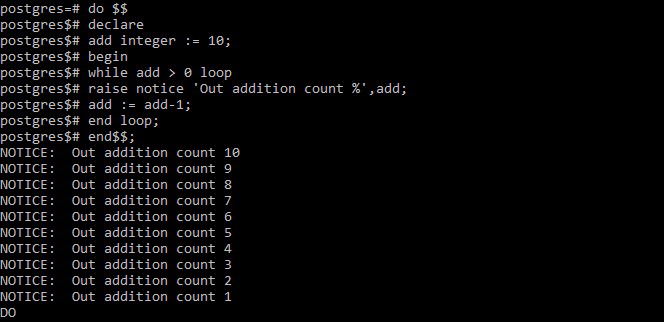# PostgreSQL – While Loops

• Last Updated : 24 Jan, 2021

PostgreSQL provides the loop statement which simply defines an unconditional loop that executes repeatedly a block of code until terminated by an exit or return statement. The while loop statement executes a block of code till the condition remains true and stops executing when the conditions become false.

The syntax of the loop statement:

```[ <<label>> ]
while condition loop
statements;
end loop;```

If we analyze the above syntax:

• Condition: If the condition is true, it executes the statements. After each iteration, the while loop again checks the condition.
• Body / Statements: Inside the body of the while loop, we need to change the values of some variables to make the condition false or null at some points. Otherwise, we will encounter the case of an infinite loop.

As the while loop tests the condition before executing the statements, it is also referred to as a pretest loop.

The following flowchart describes the while loop statement:Flowchart of While loop

Example 1:

The following code is used to raise notices while increasing the count of an arbitrary variable from 0 to 9.

```do \$\$
declare
add integer := 0;
begin
while add <10 loop
raise notice 'Out addition count %', add;
end loop;
end\$\$;```

Output:Example 2:

The following code is used to raise notices while decreasing the count of an arbitrary variable from 10 to 1.

```do \$\$
declare
add integer := 10;
begin
while add > 0 loop